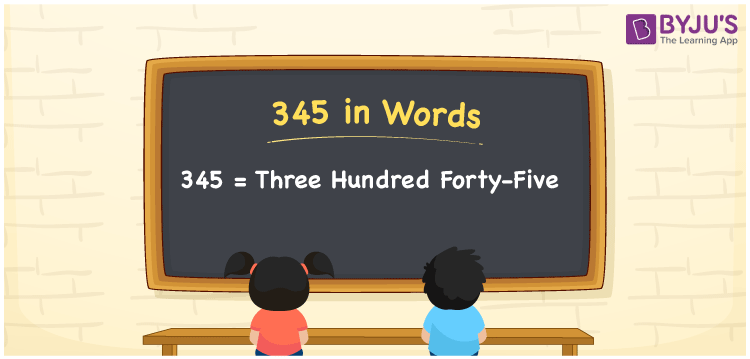# 345 in Words

345 in words is written as Three hundred forty-five. In both the International System of Numerals and the Indian System of Numerals, 345 is written as Three hundred forty-five. The number 345 is a Cardinal Number as it represents some quantity. For example, the “cost of the jacket is 345 rupees”.

 345 in Words Three hundred forty-five Three hundred forty-five in Number 345

## 345 in English Words

We write 345 in English Words using the letters of the English alphabet. Therefore, we read 345 in English as “Three hundred forty-five”.## How to Write 345 in Words?

To write 345 in words, we shall use the place value chart. In the place value chart, write 3 in the hundreds, 4 in the tens, and 5 in the ones, respectively. Now let us make a place value chart to write the number 345 in words.

 Hundreds Tens Ones 3 4 5

Thus, we can write the expanded form as

3 × Hundred + 4 × Ten + 5 × One

= 3 × 100 + 4 × 10 + 5 × 1

= 300 + 40 + 5

= 345

= Three hundred forty-five.

345 is a natural number, the successor of 344 and the predecessor of 346.

345 in words – Three hundred forty-five

• Is 345 an odd number? – Yes
• Is 345 an even number? – No
• Is 345 a perfect square number? – No
• Is 345 a perfect cube number? – No
• Is 345 a prime number? – No
• Is 345 a composite number? – Yes

## Frequently Asked Questions on 345 in Words

Q1

### How to write 345 in words?

345 in words is written as Three hundred forty-five.
Q2

### How to write 345 in the International and Indian System of Numerals?

In both, the system of numerals, 345 in words, is written as Three hundred forty-five.
Q3

### What is the successor of 345?

The successor of 345 is 346.